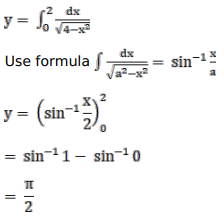# Solve this following

Question:

Mark $(\sqrt{)}$ against the correct answer in the following:

$\int_{0}^{2} \frac{d x}{\sqrt{4-x^{2}}}=?$

A. 1

B. $\sin ^{-1} \frac{1}{2}$

C. $\frac{\pi}{4}$

D. none of these

Solution: